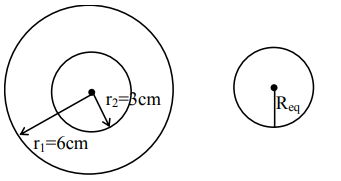# A soap bubble of radius

Question:

A soap bubble of radius $3 \mathrm{~cm}$ is formed inside the another soap bubble of radius $6 \mathrm{~cm}$. The radius of an equivalent soap bubble which has the same excess pressure as inside the smaller bubble with respect to the atmospheric pressure is $\ldots \ldots . \mathrm{cm}$.

Solution:Excess pressure inside the smaller soap bubble

$\Delta \mathrm{P}=\frac{4 \mathrm{~S}}{\mathrm{r}_{1}}+\frac{4 \mathrm{~S}}{\mathrm{r}_{2}}$  .........(I)

The excess pressure inside the equivalent soap bubble

$\Delta \mathrm{P}=\frac{4 \mathrm{~S}}{\mathrm{R}_{\mathrm{eq}}} \ldots$ (ii)

From (i) \& (ii)

$\frac{4 \mathrm{~S}}{\mathrm{R}_{\mathrm{eq}}}=\frac{4 \mathrm{~S}}{\mathrm{r}_{1}}+\frac{4 \mathrm{~S}}{\mathrm{r}_{2}}$

$\frac{1}{\mathrm{R}_{\mathrm{eq}}}=\frac{1}{\mathrm{r}_{1}}+\frac{1}{\mathrm{r}_{2}}$

$=\frac{1}{6}+\frac{1}{3}$

$\mathrm{R}_{\mathrm{eq}}=2 \mathrm{~cm}$

Ans. $2.00$